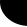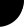HOME Electronics Directory Articles/ Tutorials eBooks About Us FORUM Links Contact UsVerilog Tutorial By Harsha Perla

Blocking and Non-blocking Assignments

Procedure assignment can be evaluated in two ways: Blocking and nonblocking assignments. If execution of other statements is blocked after evaluation of right hand side of a statement until assignment to left hand side is done, it is called blocking statement. That is, if you use a nonblocking statements in your code, right hand side of the statements to be executed without an timing delay in between will be evaluated first, and assigned later. Only if timing delay exists between two nonblocking assignments, assignment to LHS of one statements will be done before evaluation RHS of another statement. In blocking style of assignment, execution will be depending on the order in which statements are written. But in non-blocking statements, result will be independent of order. To understand it more clearly, consider these examples. "=" represents blocking and "<=" represent nonblocking in Verilog.

We will try to swap values in registers a and b, assume the value of a and b before the clock is a = 0 and b = 1.

 ```always@ (posedge clk) begin a = b; b = a; end``` `//(1)both a and b becomes 1.` ```always@ (posedge clk) begin a <= b; b <= a; end``` `//(2)a becomes 1 and b becomes 0.` ```always@ (posedge clk) fork a = b; b = a; join``` `//(3)both a and b becomes 1.` ```always@ (posedge clk) fork a <= b; b <= a; join``` `//(4)both a and b becomes 1.`

Here, code (1) and code (3) didn't give us required result. Actually in code (3) both statements should execute simultaneously because of fork--join. But, remember that executing two statements simultaneously is not possible in simulation. Evan if you write statements a = b and b = a in separate always blocks, order of execution will be depending on the simulator. Verilog permits simulators to decide which always/ initial block to to be executed first.

Code (2) and code (4) will simulate correctly.

Use non-blocking assignments to implement sequential logic and use blocking assignments to implement combinational logic. Use non-blocking assignments to implement sequential logic and use blocking assignments to implement combinational logic. (the sentence is purposefully repeated.)

We have discussed more about blocking and nonblocking assignments in "Verilog synthesis tutorial".

Prev. : begin-end and fork-join
Verilog: Table of Contents
Ch:  1. 2. 3. 4. 5. 6. 7. 8. 9. 10. 11. 12. 13.
Next: if-else statements

 Home   |    About Us   |   Articles/ Tutorials   |   Downloads   |   Feedback   |   Links   |   eBooks   |   Privacy Policy Copyright © 2005-2007 electroSofts.com. webmaster@electroSofts.com